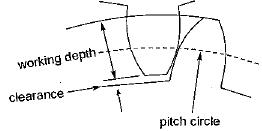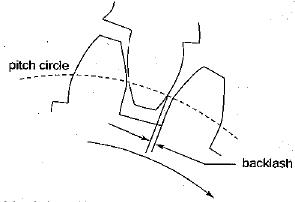Courses

# Test: Toothed Gearing - 3

## 20 Questions MCQ Test Topicwise Question Bank for Mechanical Engineering | Test: Toothed Gearing - 3

Description
This mock test of Test: Toothed Gearing - 3 for Mechanical Engineering helps you for every Mechanical Engineering entrance exam. This contains 20 Multiple Choice Questions for Mechanical Engineering Test: Toothed Gearing - 3 (mcq) to study with solutions a complete question bank. The solved questions answers in this Test: Toothed Gearing - 3 quiz give you a good mix of easy questions and tough questions. Mechanical Engineering students definitely take this Test: Toothed Gearing - 3 exercise for a better result in the exam. You can find other Test: Toothed Gearing - 3 extra questions, long questions & short questions for Mechanical Engineering on EduRev as well by searching above.
QUESTION: 1

Solution:
QUESTION: 2

### In the case of an involute toothed gear, involute starts from

Solution:

In case of involute toothed gear base circle is the circle above which profile of gear is involute and below the base circle straight line exists. Hence it is the base circle.

QUESTION: 3

### A rack is a gear of infinite

Solution:

Rack is defined as a gear of infinite diameter.

QUESTION: 4

The stub tooth is

Solution:

20° stub involute system have following features:

• Shorter addendum and shorter dedendum
• Smaller interference as compared to full depth involute
• Drive is more compact
• Stronger than full depth involute teeth
• Lower production cost as less metal must be cut away

The main draw back of this system is that the contact ratio is reduced due to shorter addendum.

QUESTION: 5

When two spur gears having involute profiles on their teeth engaged, the line of action is tangential to the

Solution:

Two important properties of an involute
(i) A normal to an involute is a tangent to the basic circle
(ii) The radius of curvature of an involute is equal to the length of the tangent to the base circle.

QUESTION: 6

For a cycloidal tooth profile, pressure angle at
I. Commencement of engagement
II. Pitch point and
III. At the end of engagement will be

Solution:

For a cycloidal tooth profile pressure angle is zero at the pitch point and becomes maximum during the engagement and disengagement.

QUESTION: 7

In gear drive, standard addendum equals

Solution:

In standard
Addendum = 1 module

QUESTION: 8

Common contact ratio of a pair of spur pinion

Solution:

Contract ratio of a pair of spur pinion and gear is usually more than 1.
Here appropriate answer is (c).

QUESTION: 9

The difference between addendum and dedendum is known as

Solution:Clearance is the amount by which the dedendum of a gear exceeds the addendum of its mating tooth.

QUESTION: 10

The difference between space width and tooth thickness is

Solution:Backlash is defined as the amount by which the width of tooth space exceeds the thickness of the engagement tooth measured along the pitch circle.

QUESTION: 11

Ratio of pitch circle diameter in millimeters to the number of teeth is known as

Solution:

Module  = D/T
where
D = Pitch circle diameter
T = Number of teeth

QUESTION: 12

In case of a gear pair with involute tooth profile, the pressure angle through out the contact is

Solution:

In involute profile of gear teeth pressure angle is constant throughout the engagement of teeth. This results in smooth running of the gear.

QUESTION: 13

Match List - I (Terms) with List - II (Definitions) and select the correct answer using the codes given below the lists:
List-I
A. Module
B. Addendum
C. Circular pitch
List-II
1. Radial distance of a tooth from the pitch circle to the top of the tooth
2. Radial distance of a tooth from the pitch circle to the bottom of the tooth
3. Distance on the circumference of the pitch circle from a point of one tooth to the corresponding point on the next tooth
4. Ratio of pitch circle diameter in mm to the number of teeth
Codes:
A B C
(a) 4 1 3
(b) 4 2 3
(c) 3 1 2
(d) 3 2 4

Solution:

Module: It is the ratio of the pitch circle diameter to the number of teeth. It is usually denoted by m. Mathematically.
Module, m = D/T
Addendum: The addendum is the radial distance between pitch and the addendum circles. Addendum indicates the height of tooth above the pitch circle.
Circular Pitch: It is the distance measured on the circumference of the pitch circle from a point of one tooth to the corresponding point on the next tooth . It is usually denoted by pc.
Mathematically,
Circular pitch
pc = πD/T
where D = Diameter of the pitch circle,
and T = Number of teeth on the wheel

QUESTION: 14

Which one of the following is the correct statement?

The consequence of a slight increase in the centre distance between two mating involute gears is that.

Solution:

Interference in involute gear can be avoided by increasing the centre distance. Interference can also be eliminated by increasing the pressure angle.

QUESTION: 15

If an imaginary circle is drawn which- by pure rolling action gives the same motion as the actual gear, what is the circle called?

Solution:

Pitch Circle: The pitch circle is the curve of intersection of the pitch surface of revolution and the plane of rotation. It is an imaginary circle that rolls without slipping with the pitch circle of a mating gear. The pitch circles of a pair of mating gears are tangent to each other.

QUESTION: 16

How does the contact ratio of helical gears compare with that of spur gears?

Solution:

P. Addendum - Gear
Q. Instantaneous centre of velocity - Linkage
R. Section modulus - Beam
S. Prime circle - Cam

QUESTION: 17

Match the items in columns I and II.
Column-I
P. Addendum
Q. Instantaneous center of velocity
R. Section modulus
S. Prime circle
Column-ll
1. Cam
2. Beam
3. Linkage
4. Gear

Solution:
QUESTION: 18

Tooth interference in an external involute spur gear pair can be reduced by

Solution:
QUESTION: 19

Two involute gears of 20° pressure angle are in mesh. The number of teeth on pinion is 20 and gear ratio is 2. If the module is 5 mm and pitch line speed is 1.2 m/s, (Assume addendum as standard and equal to one module). Then angle turned through the pinion when one pair of teeth is in mesh, will be

Solution:
QUESTION: 20

The arrangement is called bevel gearing, when two shafts are

Solution:

To have a gear drive between two intersecting shafts bevel gears are used.